# Application of OP-Amp as Inverting amplifier , Non-inverting amplifier and Voltage follower

## Application of OP-Amp as Inverting Amplifier

An OP amplifier can be operated as an inverting amplifier as shown in fig. 1.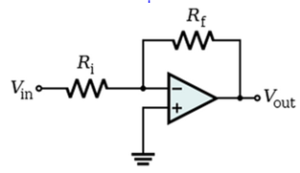Fig.1

An input signal Vin is applied through input resistor Ri to the minus input (inverting input).

The output is fed back to the same inverting input through feedback resistor Rf .

The plus input (non-inverting input) is grounded.

The resistor Rf provides the negative feedback.

Since the input signal is applied to the inverting input, the output will be inverted i.e. 180o out of phase as compared to the input. Hence, the name inverting amplifier.

Voltage Gain

An OP-Amp has infinite input impedance. This means that there is zero current at the inverting input.

If there is zero current through the input impedance, then there must be no voltage drop between the inverting and non-inverting inputs.

This means that voltage at the inverting input is zero (point A) because the other input is grounded.

The 0V at the inverting input terminal is referred to as virtual ground. The point A is said to be at virtual ground because it is at 0V but is not physically connected to the ground. This condition is shown in fig.2 (i).

Fig. 2(i)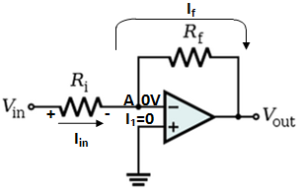Fig 2(ii)

Referring to fig.2 (ii), the current I1 to the inverting input is zero. Therefore, current Iin flowing through Ri entirely flows through feedback resistor Rf . In other words,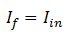Now,And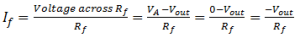Since,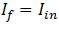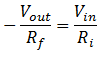Voltage gain,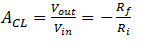The negative sign indicates that output signal is inverted as compared to input signal.

The following points may be noted about the inverting amplifier:

1. The closed-loop voltage gain ACL of an inverting amplifier is the ratio of the feedback resistance Rf to the input resistance Ri .Hence, it is independent of the OP-Amp’s internal open loop voltage gain. Thus the negative feedback stabilizes the voltage gain.
2. The inverting amplifier can be designed for unity gain if Rf = Ri
3. If Rf is some multiple of Ri , the amplifier gain is constant. Thus the inverting amplifier provides constant voltage gain.

## Application of OP-Amp as Non-Inverting Amplifier

Sometimes, we need to have an output signal of the same polarity as the input signal. In this case, the OP-Amp is connected as non-inverting amplifier as shown in fig.3.

The input signal is applied to the non-inverting input (+).

The output is applied back to the input through the feedback circuit formed by feedback resistor Rf and input resistance Ri .

Note that, resistors Rf and Ri form a voltage divider at the inverting input (-).This produces negative feedback in the circuit.

Note that R­i is grounded. Since the input signal is applied to the non-inverting input, the output signal is non-inverted i.e. the output signal will be in phase with the input signal. Hence, the name non-inverting amplifier.

Voltage Gain

If we assume that we are not at saturation, the potential at point A, is the same as Vin .

Since, the input impedance of OP-Amp is very high, all of the current that flows through Rf also flows through Ri . Keeping these things in mind, we have,

Voltage across Ri = Vin– 0;

Voltage across Rf = Vout – Vin

Now Current through Ri = Current through Rf

Or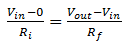Or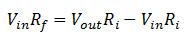Or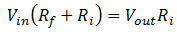Or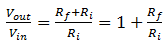Closed-loop voltage gain,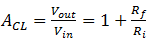The following points may be noted about the non-inverting amplifier:

1. The voltage gain of non-inverting amplifier also depends on the values of Rf & Ri
2. The voltage gain of a non-inverting amplifier can be made equal to greater than 1.
3. The voltage gain of a non-inverting amplifier will always be greater than the gain of an equivalent inverting amplifier by a value of 1.
4. The voltage gain is positive as the output signal is in phase with the input signal.

3. Voltage Follower

The voltage follower arrangement is a special case of non-inverting amplifier where all of the output voltage is fed back to the inverting input as shown in fig.4.Fig.4

It can be noted that Ri and Rf are removed from the non-inverting amplifier and the output of the amplifier is shorted to the inverting input.

The voltage gain for the voltage follower is calculated as follows:Thus the closed-loop voltage gain of the voltage follower is 1.

The most important feature of the voltage follower is that it has a very input impedance. This makes it nearly ideal buffer amplifier to be connected between high-impedance sources and low-impedance loads.